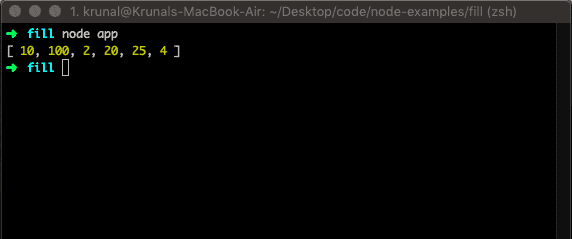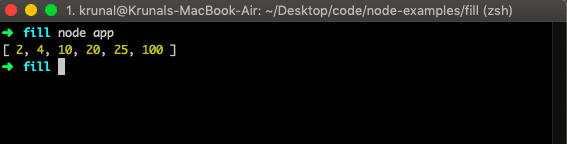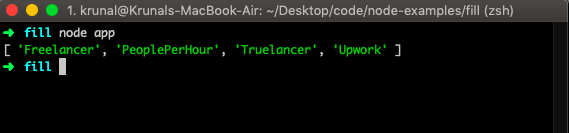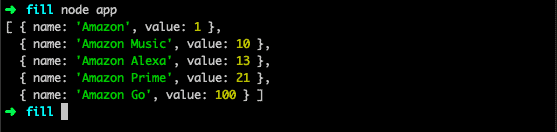# JavaScript Array Sort() Method

JavaScript array sort() is a built-in method that sorts all the array’s elements in place and returns that array. It accepts parameters like functions and will sort an array based on that function logic. The default sort order is built on converting the item into strings and comparing their UTF-16 code unit values sequences.

### Syntax

``array.sort(compareFunction)``

### Parameters

The compareFunction() parameter is an optional argument. The function defines an alternative sort order, and it should return a negative, zero, or positive value, depending on the arguments.

### Example

Let us take a simple example without a compare function. First, create an app.js file and add the code.

``````let points = [4, 10, 100, 25, 2, 20];

points.sort();

console.log(points);``````

Run the above file by typing the node app command in the terminal. See the output, and it is not what you expected.

OutputAgain, it does not merely put the values in ascending order. By default, the array sort() function sorts the values as strings in alphabetical and ascending order.

### Example 2

Let us pass the compareFunction and change the default sort order.

``````let points = [4, 10, 100, 25, 2, 20];

const sortAsc = (a, b) => a - b;

points.sort(sortAsc);

console.log(points);``````

So, here we are sorting the values in Ascending order, and we have written one JS function to do that. Run the file.

OutputWe can do the Descending order also.

``````let points = [4, 10, 100, 25, 2, 20];

const sortAsc = (a, b) => b - a;

points.sort(sortAsc);

console.log(points);``````

If the compareFunction() is not supplied, all non-undefined array elements are sorted by converting them to strings and comparing strings in UTF-16 code units order.

``````let freelance = ['Upwork', 'Freelancer', 'Truelancer', 'PeoplePerHour'];

freelance.sort();

console.log(freelance);``````

Output## Sorting an array of objects

To sort an array of objects by a property value in JavaScript, you must provide a compare function to the sort() method that compares the property values of two objects.

``````let apps = [
{ name: 'Amazon', value: 1 },
{ name: 'Amazon Prime', value: 21 },
{ name: 'Amazon Music', value: 10 },
{ name: 'Amazon Go', value: 100 },
{ name: 'Amazon Alexa', value: 13 }
];
apps.sort((a, b) => a.value-b.value);

console.log(apps);``````

Here, we have used the arrow function. Now, it will sort the Ascending order based on the values.

Output## Sorting non-ASCII characters and localeCompare()

For sorting strings with non-ASCII characters, i.e., strings with accented characters like these (e, é, è, a, ä, etc.),

The strings from other languages apart from English: use the String.localCompare() method. The String.localCompare() function can compare those characters so they appear in the right order.

``````let words = ['réservé', 'premier', 'cliché', 'communiqué', 'café', 'adieu'];

words.sort(function (a, b) {
return a.localeCompare(b);
});

console.log(words);``````

Output

``[ 'adieu', 'café', 'cliché', 'communiqué', 'premier', 'réservé' ]``

## Sorting an array of numbers

JavaScript array sort() method arranges the array elements in some order. By default, it sorts the elements as strings in ascending order. Likewise, by default, the sort() function sorts values as strings.

This works well for strings (“Apple” comes before “Banana”).

However, if numbers are sorted as strings, “25” is bigger than “100” because “2” is bigger than “1”.

Because of this, the sort() method will produce incorrect results when sorting numbers.

``````let points = [11, 19, 21, 18, 46, 10];

points.sort((a, b) => a - b);

console.log(points)``````

Output

``[ 10, 11, 18, 19, 21, 46 ]``

When a sort() function compares two values, it sends the values to the compare function and sorts the values according to the returned, which are the negative, zero, and positive.

If the result is negative, then a is sorted before b.

If the result is positive, then b is sorted before a.

If the result is 0, then no changes are made with the sort order of the two values.

## Sorting an Array in random order

We can use Javascript Math.random() function to get the random results.

``````let points = [11, 19, 21, 18, 46, 10];

points.sort(() => 0.5 - Math.random());

console.log(points)``````

Output

``````node app
[ 10, 11, 18, 19, 21, 46 ]
node app
[ 11, 46, 10, 19, 18, 21 ]
node app
[ 10, 21, 46, 19, 18, 11 ]
node app
[ 21, 10, 18, 46, 19, 11 ]
node app
[ 10, 46, 11, 19, 21, 18 ]
node app
[ 10, 21, 19, 46, 18, 11 ]
node app
[ 10, 19, 21, 11, 18, 46 ]``````

From the output, you can see that every time we run the node.js file, we will get a different sorted random output.

## Sorting the array of objects by a specified property

To sort an array of objects by a property value in JavaScript, you must provide a compare function to the sort() method that compares the property values of two objects.

``````let employees = [
{ name: 'Olivia', salary: 90000, age: 29 },
{ name: 'Taylor', salary: 75000, age: 32 },
{ name: 'Mea', salary: 85000, age: 35 }
];

employees.sort((x, y) => x.salary - y.salary);
console.log(employees)``````

Output

``````[
{ name: 'Taylor', salary: 75000, age: 32 },
{ name: 'Mea', salary: 85000, age: 35 },
{ name: 'Olivia', salary: 90000, age: 29 }
]``````

The above code example is similar to the example of sorting the array of numbers in ascending order. The only difference is that it compares the salary property of two objects instead.

That’s it.

### 1 thought on “JavaScript Array Sort() Method”

1.thanks for the guide